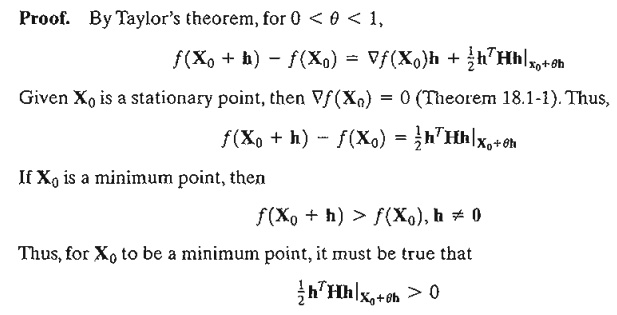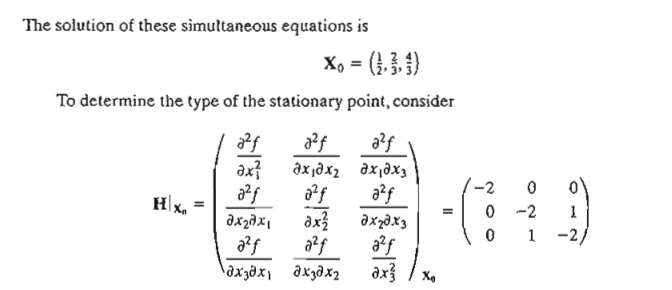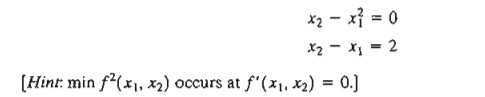Home | | Resource Management Techniques | Unconstrained Problems -Classical Optimization Theory

# Unconstrained Problems -Classical Optimization Theory

An extreme point of a function f(X) defines either a maximum or a minimum of the functions

UNCONSTRAINED PROBLEMS

An extreme point of a function f(X) defines either a maximum or a minimum of theFigure 18.1 illustrates the maxima and minima of a single-variable function f(x) over the interval [a,b]. The points x1, x2, x3, x4, and x6 are all extrema of f(x), with xl, x3, and x6 as maxima and x2 and x4 as minima. Becausef(x6) is a global or absolute maximum, and f(x1) and f(x3) are local or relative maxima. Similarly,f(x4) is a local minimum and f(x2) is a global minimum.Although x1 (in Figure 18.1) is a maximum point, it differs from remaining local maxima in that the value of f corresponding to at least one point in the neighborhood of x1 equals f(x1) In this respect, x1 is a weak maximum, whereas x3 and x6 are strong maxima. In general, for h as defined earlier, X o is a weak maximum if f(X o + h) ≤ f(X 0) and a strong maximum if f(X o + h) < f(X o).

In Figure 18.1, the first derivative (slope) of f equals zero at all extrema. However, this property is also satisfied at inflection and saddle points, such as x5. If a point with zero slope (gradient) is not an extremum (maximum or minimum), then it must be an inflection or a saddle point.

1. Necessary and Sufficient Conditions

This section develops the necessary and sufficient conditions for an n-variable function f(X) to have extrema. It is assumed that the first and second partial derivatives of f(X) are continuous for all X.We show by contradiction that Ñf(X o) must vanish at a minimum point Xo. For suppose it does not, then for a specific j the following condition will hold.This result contradicts the assumption that X o is a minimum point. Thus, Ñf(X o) must equal zero. A similar proof can be established for the maximization case.

Because the necessary condition is also satisfied for inflection and saddle points, it is more appropriate to refer to the points obtained from the solution of Ñf(Xo) = 0 as stationary points. The next theorem establishes the sufficiency conditions for X o to be an extreme point.

Theorem 18.1-2. A sufficient condition for a stationary point X o to be an extremum is that the Hessian matrix H evaluated at X o satisfy the following conditions:

i.            H is positive definite if Xois a minimum point.

ii.            H is negative definite I fXo is a maximum point.

Proof. By Taylor's theorem, for 0 < q < 1,Given that the second partial derivative is continuous, the expression  ½  hTHh must have the same sign at both Xo and Xo + qh. Because hTHhl Xo defines a quadratic form (see Section 0.3 on the CD), this expression (and hence hTXh|Xo+qh) is positive if and only if, H|x0 is positive-definite. This means that a sufficient condition for the stationary point X o to be a minimum is that the Hessian matrix, H, evaluated at the same point is positive-definite. A similar proof for the maximization case shows that the corresponding Hessian matrix must be negative-definite.The principal minor determinants of H|xo have the values -2,4, and -6, respectively. Thus, as shown in Section D.3, HIXu is negative-definite and Xo = (1/2, 2/3, 4/3) represents a maximum point.

In general, if H|x0 is indefinite, Xo must be a saddle point. For nonconclusive cases, Xo mayor may not be an extremum and the sufficiency condition becomes rather involved, because higher-order terms in Taylor's expansion must be considered.

The sufficiency condition established by Theorem 18.1-2 applies to single-variable functions as follows. Given that yo is a stationary point, then

i.            yo is a maximum if f” (yo)  < 0.

ii.            yo is a minimum if f" (yo)  > 0.

If f” (y0) = 0, higher-order derivatives must be investigated as the following theorem requires.Theorem 18.1-3. Given yo, a stationary point of f(y), if the first (n  - 1) derivatives are zero and f(n)(yo) != 0, then

i.            If n is odd, yo is an inflection point.

ii.            If n is even then yo is a minimum if f(n)(yo> 0 and a maximum if f(n)(yo< 0.

Example 18.1-2

Figure 18.2 graphs the following two functions:3. Verify that the functionhas the stationary points (0, 3, 1), (0, 1, -1), (1,2,0), (2, 1, 1), and (2, 3, -1). Use the sufficiency condition to identify the extreme points.

*4. Solve the following simultaneous equations by converting the system to a nonlinear objective function with no constraints.5. Prove Theorem 18.1-3.

2. The Newton-Raphson Method

In general, the necessary condition equations, Ñ f(X) = 0, may be difficult to solve numerically. The Newton-Raphson method is an iterative procedure for solving simultaneous nonlinear equations.

Consider the simultaneous equationsThe idea of the method is to start from an initial point X o and then use the equation above to determine a new point. The process continues until two successive points, X k and X k +1 are approximately equal.

A geometric interpretation of the method is illustrated by a single-variable function in Figure 18.3. The relationship between xk and xk+1 for a single-variable function f(x) reduces toFIGURE 18.3

Illustration of the iterative process in the Newton-Raphson methodThe figure shows that xk+1 is determined from the slope of f(x) at xk where tan q = f' (xk).

One difficulty with the method is that convergence is not always guaranteed unless the function f is well behaved. In Figure 18.3, if the initial point is a, the method will diverge. In general, trial and error is used to locate a "good" initial point.

Example 18.1-3

To demonstrate the use of the Newton-Raphson method, consider determining the stationary points of the functionTo determine the stationary points, we need to solveThe method converges to x = 1.5. Actually, f(x) has three stationary points at x = 2/3, x = 13/12, and x = 3/2. The remaining two points can be found by selecting different values for initial xo. In fact, xo = .5 and xo = 1 should yield the missing stationary points.

Excel Moment

Template excelNR.xls can be used to solve any single-variable equation. It requires entering f(x)/f' (x) in cell C3. For Example 18.1-3, we enterThe variable x is replaced with A3. The template allows setting a tolerance limit Δ, which specifies the allowable difference between xk and xk+1 that signals the termination of the iterations. You are encouraged to use different initial xo to get a feel of how the method works.

In general, the Newton-Raphson method requires making several attempts before "all" the solutions can be found. In Example 18.1-3, we know beforehand that the equa-tion has three roots. This will not be the case with complex or multi-variable functions, however.

PROBLEM SET 18.1B

1.     Use NewtonRaphson.xls to solve Problem I(c), Set I8.la.

2.     Solve Problem 2(b), Set I8.la by the Newton-Raphson method.

Study Material, Lecturing Notes, Assignment, Reference, Wiki description explanation, brief detail
Operations Research: An Introduction : Classical Optimization Theory : Unconstrained Problems -Classical Optimization Theory |